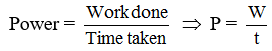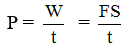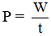Ex﻿

# 3. Work and Energy

#### Power & its Unit

Definition : Power is defined as the rate of doing workIn other words, power is the work done per unit time, power is a scalar quantity.
Since W = F.S therefore= F × V = force × velocity
Unit of power : The S.I. unit of power is watt and it is the rate of doing work at 1 joule per second.
1 watt is equal to :1 kilowatt = 1 kW = 1000 W
1 Horse power = 1 H.P. = 746 W
Example : 8
A machine raises a load of 750 N through a height of 15 m in 5s. Calculate :
(i) the work done by the machine.
(ii) the power at which the machine works.
Solution. (i) Work done is given by W = F.s
Here F = 750 N; s = 15 m
∴ W = 750 × 15 = 11250 J
= 11.250 kJ
(ii) Now, power of the machine is given byHere, W = 11250 J; t = 5 s
∴ Power P = 11250J/5s = 2250 W = 2.250 kW

If you want to give information about online courses to other students, then share it with more and more on Facebook, Twitter, Google Plus. The more the shares will be, the more students will benefit. The share buttons are given below for your convenience.
×

#### NTSE Physics (Class X)

• Electricity
• Magnetic Effect of Curent
• Light Reflection
• Light Refraction
• Human Eye & Colourful World
• Source of Energy
• Motion

#### NTSE Physics (Class IX)

• Force & laws of motion
• Work and Energy
• Gravitation
• Sound

SHOW CHAPTERS